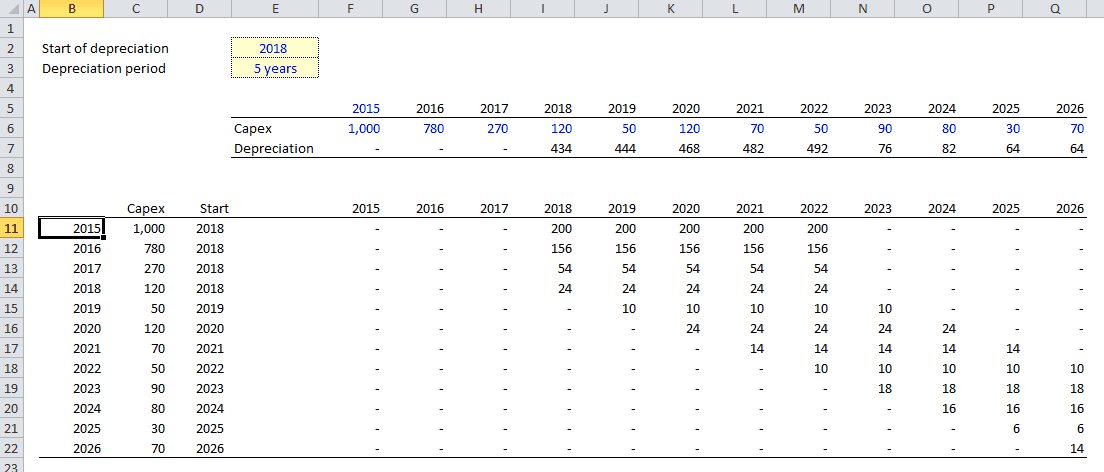top of page

## Coding

Depreciation

The following methodology describes how to model linear depreciation.

Excel modeling

1. Create two columns on the left: in the first one specify the first year of the model (assuming the model is annual), in the second one return the corresponding capital expenditure of the year (you can use a combination of INDEX and MATCH to do so)

2. Add an additional column that returns the applicable depreciation period for each year

3. Add another column that specifies when depreciation starts for each capital expenditure (the maximum between the start of depreciation for the project and the year in which the capital expenditure occured)

4. Using the IF function, make sure depreciation only starts when the current year is superior or equal to the depreciation start year that you calculated in 3

5. The annual depreciation is the minimum between the remaining undepreciated value and the gross asset value (its historical cost) divided by the number of years over which the asset must be depreciatedbottom of page